MP Board Class 6th Maths Solutions Chapter 11 Algebra Ex 11.1

Question 1.
Find the rule which gives the number of matchsticks required to make the following matchstick patterns. Use a variable to write the rule.
(a) A pattern of letter T as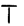(b) A pattern of letter Z as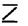(c) A pattern of letter U as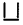(d) A pattern of letter V as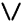(e) A pattern of letter E as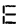(f) A pattern of letter S as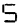(g) A pattern of letter A as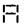Solution:
(a) Number of matchsticks required to make one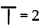∴ Number of matchsticks required to
make a pattern of letter T as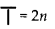(b) Number of matchsticks required to make one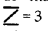∴ Number of matchsticks required to make a pattern of letter Z as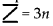(c) Number of matchsticks required to make one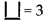∴Number of matchsticks required to make a pattern of letter U as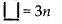(d) Number of matchsticks required to make one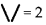Number of matchsticks required to make a pattern of letter V as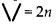(e) Number of matchsticks required to make one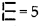∴ Number of matchsticks required to make a pattern of letter E as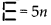(f) Number of matchsticks required to make one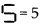∴ Number of matchsticks required to make a pattern of letter S as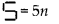(g) Number of matchsticks required to make one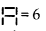∴ Number of matchsticks required to make a pattern of letter A as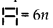Question 2.
We already know the rule for the pattern of letters L, C and F. Some of the letters from Q.1 (given above) give us the same rule as that given by L. Which are these? Why does this happen?
Solution:
Part (a) & (d) i.e., letter T and V have same rule as that given by L.
Because the number of matchsticks required in each of them is 2.

Question 3.
Cadets are marching in a parade. There are 5 cadets in a row. What is the rule which gives the number of cadets, given the number of rows? (Use n for the number of rows.)
Solution:
Let number of rows = n
Number of cadets in each row = 5
Therefore, total number of cadets = 5MQuestion 4.
If there are 50 mangoes in a box, how will you write the total number of mangoes in terms of the number of boxes? (Use b for the number of boxes.)
Solution:
Let number of boxes = b
Number of mangoes in each box = 50
Therefore, total number of mangoes = 50b

Question 5.
The teacher distributes 5 pencils per student. Can you tell how many pencils are needed, given the number of students? (Use s for the number of students.)
Solution:
Let number of students = s
Number of pencils distributed to each student = 5
Therefore, total number of pencils needed = 5s

Question 6.
A bird flies 1 kilometer in one minute. Can you express the distance covered by the bird in terms of its flying time in minutes? (Use t for flying time in minutes.)
Solution:
Let flying time of the bird be t minutes.
Distance covered by the bird in 1 minute = 1 km
∴ Distance covered by the bird in t minutes = 1 × t km = t kmQuestion 7.
Radha is drawing a dot Rangoli (a beautiful pattern of lines joining dots) with chalk powder. She has 9 dots in a row. How many dots will her Rangoli have for r rows? How many dots are there if there are 8 rows? If there are 10 rows?
Solution:
Number of dots in each row = 9
Number of rows = r
Therefore, total number of dots = 9r
When there are 8 rows, then number of dots = 9 × 8 = 72
When there are 10 rows, then number of dots = 9 × 10 = 90

Question 8.
Leela is Radha’s younger sister. Leela is 4 years younger than Radha. Can you write Leela’s age in terms of Radha’s age? Take Radha’s age to be x years.
Solution:
Since, Leela is 4 years younger than Radha.
Therefore, Leela’s age = (x – 4) years.

Question 9.
Solution:
Number of laddus gave away = l
Number of remaining laddus = 5
∴ Total number of laddus = (l + 5)Question 10.
Oranges are to be transferred from larger boxes into smaller boxes. When a large box is emptied, the oranges from it fill two smaller boxes and still 10 oranges remain outside. If the number of oranges in a small box are taken to be x, what is the number of oranges in the larger box?
Solution:
Number of oranges in a small box = x
Number of smaller boxes = 2
Therefore, total number of oranges in smaller boxes = 2x
Number of remaining oranges = 10
Thus, number of oranges in the larger box = 2x + 10

Question 11.
(a) Look at the following matchstick pattern of squares (see fig.).The squares are not separate. Two neighbouring squares have a common matchstick.
Observe the patterns and find the rule that gives the number of matchsticks in terms of the number of squares. (Hint: If you remove the vertical stick at the end, you will get a pattern of Cs.)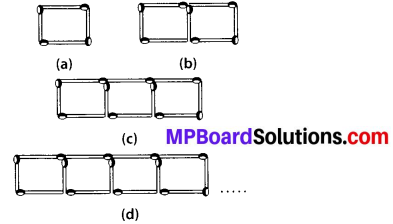(b) The given figure gives a matchstick pattern of triangles. As in Exercise 11 (a) above, find the general rule that gives the number of matchsticks in terms of the number of triangles.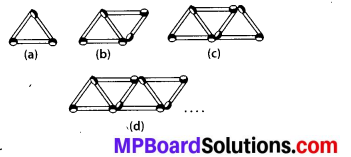Solution:
(a)If we remove 1 matchstick from each figure, then they make multiples of 3, i.e., 3, 6, 9, 12, …………
So the required equation = 3x + 1, where x is number of squares.

(b)If we remove 1 matchstick from each figure, then they make multiples of 2, i.e., 2, 4, 6, 8, ………..
So the required equation = 2x + 1, where x is number of triangles.